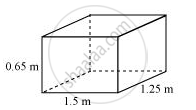# A plastic box 1.5 m long, 1.25 m wide and 65 cm deep, is to be made. It is to be open at the top. Ignoring the thickness of the plastic sheet, determine - Mathematics

A plastic box 1.5 m long, 1.25 m wide and 65 cm deep, is to be made. It is to be open at the top. Ignoring the thickness of the plastic sheet, determine:

(i) The area of the sheet required for making the box.

(ii) The cost of sheet for it, if a sheet measuring 1 m2 costs Rs 20.

#### SolutionIt is given that, length (l) of box = 1.5 m

Breadth (b) of box = 1.25 m

Depth (h) of box = 0.65 m

(i) Box is to be open at top.

Area of sheet required

= 2lh + 2bh + lb

= [2 × 1.5 × 0.65 + 2 × 1.25 × 0.65 + 1.5 × 1.25] m2

= (1.95 + 1.625 + 1.875) m2 = 5.45 m2

(ii) Cost of sheet per m2 area = Rs 20

Cost of sheet of 5.45 m2 area = Rs (5.45 × 20)

= Rs 109

Concept: Surface Area of a Cuboid
Is there an error in this question or solution?

#### APPEARS IN

NCERT Class 9 Maths
Chapter 13 Surface Area and Volumes
Exercise 13.1 | Q 1 | Page 213